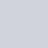# WACC Calculator | Discount Rate Estimation

This model calculates the Weighted Average Cost of Capital (WACC) which can be used as discount rate for any DCF calculation.Financial Model, General Excel Financial Models DCF Model, Discount Rate, Excel, Free Financial Model Templates, NPV (Net Present Value)

This Excel model calculates the Weighted Average Cost of Capital (WACC) or discount rate which is used when building a DCF model to discount future cash flows to firm to their present value.

The weighted average cost of capital has three main components: The cost of equity, the cost of debt and the weighting factor. The model contains the WACC formula and allows you to systematically work through the required financial parameters to fine-tune and justify your discount rate by calculating the WACC.

The discount rate is a measure of a company’s risk and the required opportunity cost to compensate investors to carry such. The higher the discount rate, the lower the present value of future cash flows. Given the discount rate is one of the major drivers in almost any DCF valuations, a careful analysis is required.

## Reviews

•Excellent WACC Calculator

Great spreadsheet, easy to use and nice layout. Thanks for the free spreadsheet.

1 of 2 people found this review helpful.

Help other customers find the most helpful reviews

Did you find this review helpful?

## Get these templates too for a discount!

### Renewable Energy Template Bundle

Price: \$140.00

### Manufacturing Financial Model Templates Bundle

Price: \$149.95

### Sports Financial Model Bundle

Price: \$140.00